# Quansulting the oracle

Welcome to Introduction to Quantum Computing. I am your guide, Associate Professor Chris Ferrie, a researcher in the UTS Centre for Quantum Software and Information. This is Lecture 7. It would probably be a good idea to have read the previous Lectures before continuing.

`What did you learn last week?Last week you were introduced to your first two quantum protocols: superdense coding and quantum teleportation. These clearly utilise entanglement as a resource to achieve a task not possible with classical bits.What will you learn this week?We will continue the theme of quantum advantages this week by studying the Deutsch-Jozsa algorithm. This algorithm also deterministically solves a problem which is impossible to solve via classical means with the same number of resources. This will be your first taste of a computational speed-up provided by quantum computers.What will you be able to do at the end of this week 5?At the end of this module, you should be able to answer these questions:What problem does the Deutsch-Jozsa algorithm solve?What is query complexity and the difference between quantum and classical?What is the quantum/classical query complexity of the Deutsch problem?`

# What the f is a balanced function?

The Deutsch-Jozsa algorithm solves a specific computational problem. It’s not an obvious, or even useful, problem. However, the purpose of the algorithm was only to show a computational advantage for some problem, and that is quite remarkable. So, you’ll have to bear with me a bit while we step through the argument — I don’t want to hear any of those “but when will this ever be useful” comments! It won’t ever be useful — that’s not the point.

The problem the Deutsch-Jozsa algorithm solves concerns the properties of Boolean functions. A Boolean function f is a mapping from many bits to a single bit. Since everything is finite, the alternatives can be enumerated. So, let’s look at the simplest two cases. The first is a function that maps a bit to a bit. There are four such functions: the identity, the NOT, the zero, and the one.

We can categorise these functions in many ways. Above we have identified one way to distinguish functions in terms of whether the output is constant (either all 0 or all 1) or balanced (same number of 0’s and 1’s as output). This categorisation is not exhaustive if we increase the number of input bits. For example, here are all sixteen possible two bits to one bit functions.

You can immediately start to imagine that as we increase the number of input bits, the vast majority of potential functions are going to neither be constant nor balance. These properties imply a restrictive structure on the function. But suppose anyway that we do restrict ourselves to functions of one of these types and ask: how many inputs would we need to check before we were certain the function we have is constant or balanced? This is called the Deutsch problem.

The answer, we will see, depends on whether you ask the questions classically or quantumly!

# Consulting the oracle

Suppose we are given a black-box implementation of an unknown Boolean function, f. Calling the function on a single input, say f(0,0), is called a query and the function is called an oracle. How many times we need to call the function to solve some problem is the query complexity of the problem. Calling the function one input at a time — similar to how I wrote each entry of the tables above — is called classical querying. Obviously there is going to be quantum query complexity, but let’s first find out what the classical query complexity of the constant vs. balanced function problem is.

It’s illustrative to start with an example. Let’s take the two-bit example above and keep only the constant and balanced functions.

If we start with the input 00 and get 0, we eliminate half the functions, namely those with f(0,0) = 1. But of the remaining four functions, one is constant and the other three are balanced. So, we cannot solve the problem with a single query. Obviously for the first query, it didn’t matter which input we tried. But now we have some information. However, no matter what next input we try, we are equally likely to get 0 or 1. So, without loss of generality, suppose we try 01. If we find f(0,1) = 1, we are done, we know the function f is not constant. However, if we find f(0,1) = 0, we are left with one constant and one balanced function that are consistent with both of the first two queries. For these two functions, the next query must distinguish them. Try to convince yourself that if the outcomes worked out differently, we would never need to query all four inputs — two or three always suffices.

Imagine that you are querying a general Boolean function that takes n bits. As soon as you find two inputs with different outputs you know the function is not constant. What is the most number of inputs a balanced function can have the same output on? Exactly half the total number of inputs, by definition! For n bits, there are 2ⁿ possible inputs. So, in the worst case, you would need half the total inputs plus one, or 2ⁿ⁻¹ + 1, queries before you were certain that the unknown function was constant or balanced.

How about quantum queries — how many do you think you will need?

# Quansulting the oracle

What does it even mean to quantumly consult an oracle? It’s actually much simpler than you think. We just apply the Boolean function to the computational basis labels. If x is an n-bit string representing an n-qubit state, then we can try |x⟩⟼|f(x)⟩. However, if f is a constant function, this is not reversible and hence not unitary. The solution is copy the input and add the output to a new “scratch” qubit: |x⟩|y⟩⟼|x⟩|y⊕f(x)⟩. Now, before you get all “but no-cloning!” on me, remember that cloning a basis is perfectly fine.

As an explicit example, we can take the simplest single-qubit example and find the exact circuit for each of the four Boolean functions.

Great! On the other hand, why are we bothering with simple classical logic — where’s all the superposition and entanglement?!

# Why NOT that qubit?

Recall that the Hadamard gate was able to turn the |000…⟩ state to the superposition of all basis states |+++…⟩. But since each quantum oracle maps the computational basis states to other computational basis states, the oracles don’t change the state |+++…⟩. We need a way to change the coefficients — and do so in a way that distinguishes constant and balanced oracles.

There is something special about that scratch qubit, since it contains the value of f(x). So, let’s start there. Instead of using the |+⟩ state, let’s try the |−⟩ state. Why? Well, (−1) is as far away a coefficient as (+1) after all! Let’s try the simple single-qubit example again, and put the scratch qubit in the |−⟩ state.

Now, if we place the querying qubit in superposition, we have the following (ignoring global phases).

Notice that the state of the first register is perfectly distinguishable. We need only bring those two possibilities back to the computational basis and we know how to do that with the Hadamard gate. The full protocol in circuit form is as follows. See if you can figure out (using the fact the the output is the |1⟩ state) if the Oracle function is constant or balanced.

Note that this only requires a single quantum query as opposed to the two classical queries necessary to solve the problem.

# More fine qubits, same great price!

The Deutsch-Jozsa algorithm generalises beyond one-bit functions to an arbitrary number of bits. What’s incredible, though, is that still only a single quantum query is sufficient to determine whether the function is constant or balanced. The generalisation is easiest to visualise, having seen the one-bit circuit above, by looking at the circuit.

The circuit details the entire algorithm, except of course the oracle function, which is meant to be “hidden”. The output of the one-bit version was |0⟩ if the function is constant. For n bits, the output will be |0000…⟩ if the function is constant, and anything else if the function is balanced. In this case, we can see from the output that the hidden function is indeed balanced since the result of the measurement is not |0000⟩.

Your homework this week is to analyse this circuit and verify it works as advertised. You have all the tools you need. Get to it!

Why not watch David Deutsch himself explain the algorithm himself?

The Wikipedia article Deutsch-Jozsa algorithm also goes over the basics and history.

Microsoft’s Quantum Katas contains a tutorial and kata on the Deutsch-Jozsa algorithm.

--

--

--

## More from Chris Ferrie

Quantum theorist by day, father by night. Occasionally moonlighting as a author. csferrie.com

Love podcasts or audiobooks? Learn on the go with our new app.

## Challenges of running gRPC services in production (part 2)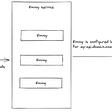## Announcing Android Full Release and New Version of Decentr Browser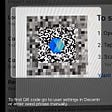## Cloud partner for successful conversion to My Data System (featuring MyData demonstration support…## Practical Tools with Python — Google Elevation API## ESP and MongoDB, sitting in a tree.## DEDICATED TEAM MODEL — Working with a Dedicated Team (Interview with Viorel Costea…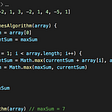## How To Install PHP On Windows OS — 6 Steps To Follow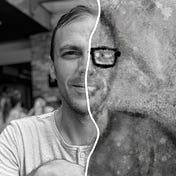## Chris Ferrie

Quantum theorist by day, father by night. Occasionally moonlighting as a author. csferrie.com

## The Quantum World Part 1: Schrodinger’s CAT!## The Case for an Annual “Quantum Games” Competition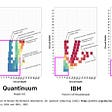## Quantum Computing with Alberto García